# Precalculus : Express a Vector in Component Form

## Example Questions

### Example Question #1 : Express A Vector In Component Form

Express the following vector in component form: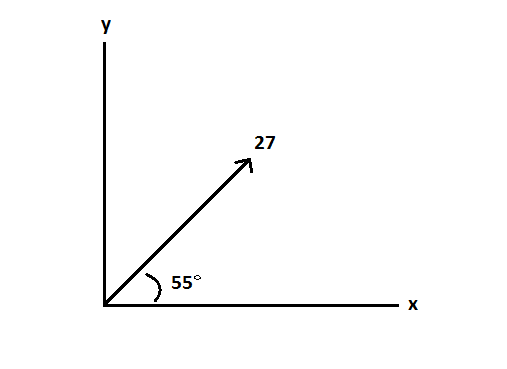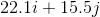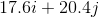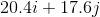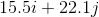Explanation:

When separating a vector into its component form, we are essentially creating a right triangle with the vector being the hypotenuse.

Therefore, we can find each component using the cos (for the x component) and sin (for the y component) functions: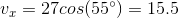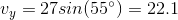We can now represent these two components together using the denotations i (for the x component) and j (for the y component).

### Example Question #2 : Express A Vector In Component Form

Find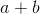, then find its magnitude.andare both vectors.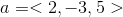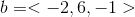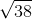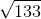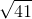Explanation:

In vector addition, you simply add each component of the vectors to each other.

x component: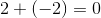.

y component: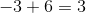.

z component: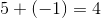.

The new vector is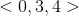.

To find the magnitude we use the formula,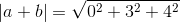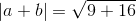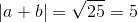Thus its magnitude is 5.

### Example Question #3 : Express A Vector In Component Form

Find the component form of the vector with

initial point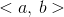and

terminal point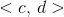.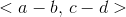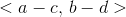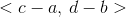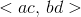Explanation:

To find the vector in component form given the initial and terminal points, simply subtract the initial point from the terminal point.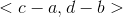### Example Question #4 : Express A Vector In Component Form

Find the component form of the vector with

initial point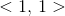and

terminal point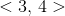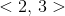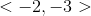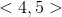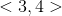Explanation:

To find the vector in component form given the initial and terminal points, simply subtract the initial point from the terminal point.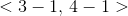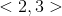### Example Question #5 : Express A Vector In Component Form

A bird flies 15 mph up at an angle of 45 degrees to the horizontal.  What is the bird's velocity in component form?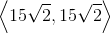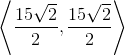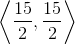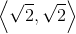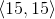Explanation:

Write the formula to find both the x and y-components of a vector.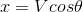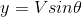Substitute the value of velocity and theta into the equations.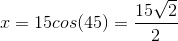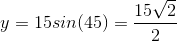The vector is:### Example Question #6 : Express A Vector In Component Form

Write this vector in component form: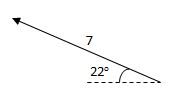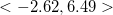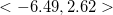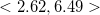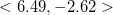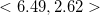Explanation:

In order to find the horizontal component, set up an equation involving cosine with 7 as the hypotenuse, since the side in the implied triangle that represents the horizontal component is adjacent to the 22-degree angle: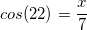First, find the cosine of 22, then multiply by 7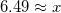To find the vertical component, set up an equation involving sine, since the side in the implied triangle that represents the vertical component is opposite the 22-degree angle: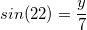First, find the sine of 22, then multiply by 7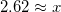We are almost done, but we need to make a small adjustment. The picture indicates that the vector points up and to the left, so the horizontal component, 6.49, should be negative:### All Precalculus Resources